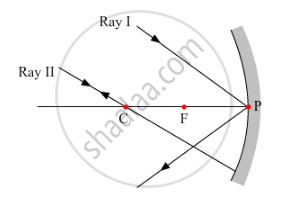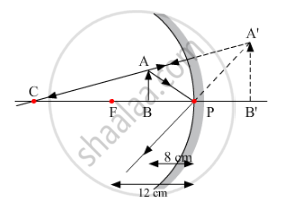# To Construct Ray Diagrams, Two Rays of Light Are Generally So Chosen that It is Easy to Determine Their Directions After Reflection from a Mirror. - Science

To construct ray diagrams, two rays of light are generally so chosen that it is easy to determine their directions after reflection from a mirror. Choose two such rays and state the path/direction of these rays after reflection from a concave mirror. Use these two rays to find the position and nature of the image of an object placed at a distance of 8 cm from a concave mirror of focal length 12 cm.

#### Solution

The two rays chosen to construct a ray diagram are shown in the ray diagram given below.(i) Ray I:- When the ray is incident obliquely to the principle axis towards the pole of the concave mirror, the angle at which it reflects back is equal to the angle of incidence.

(ii) Ray II:- When the incident ray passes through the centre of curvature, the light, after reflection from the concave mirror, reflects back along the same path.The image formed is virtual, erect and magnified. It is formed behind the mirror.

Concept: Concave Mirror
Is there an error in this question or solution?